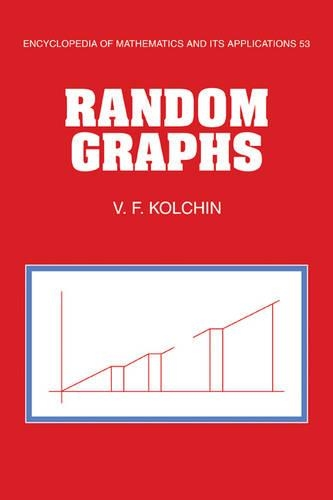•# Random Graphs - Encyclopedia of Mathematics and its Applications (Paperback)

(author)
£39.99
Paperback 268 Pages / Published: 17/09/2009
• We can order this

Usually dispatched within 15 working days

This book is devoted to the study of classical combinatorial structures such as random graphs, permutations, and systems of random linear equations in finite fields. The author shows how the application of the generalized scheme of allocation in the study of random graphs and permutations reduces the combinatorial problems to classical problems of probability theory on the summation of independent random variables. He concentrates on research by Russian mathematicians, including a discussion of equations containing an unknown permutation and a presentation of techniques for solving systems of random linear equations in finite fields. These results will interest specialists in combinatorics and probability theory and will also be useful in applied areas of probabilistic combinatorics such as communication theory, cryptology, and mathematical genetics.

Publisher: Cambridge University Press
ISBN: 9780521119689
Number of pages: 268
Weight: 380 g
Dimensions: 234 x 156 x 14 mm